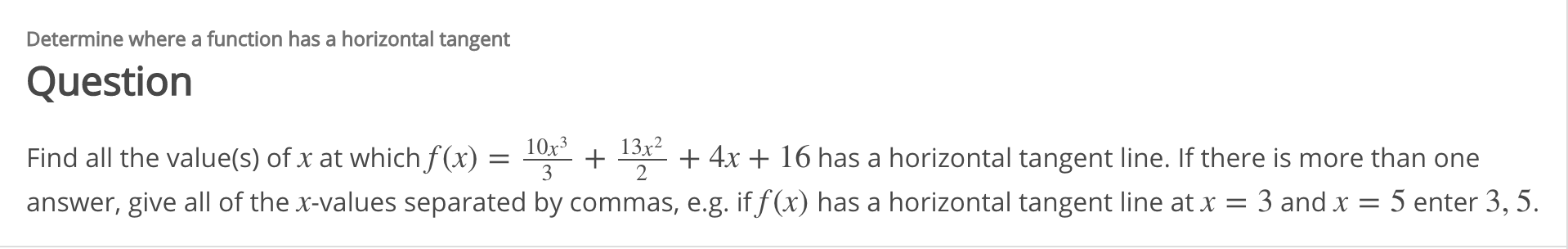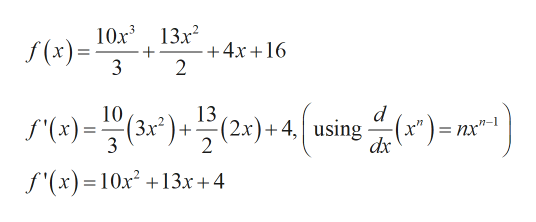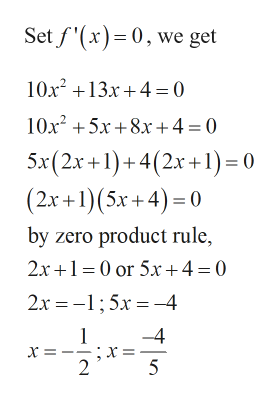# Determine where a function has a horizontal tangentQuestion10x313x2Find all the value(s) of x at which f(x) =answer, give all of the x-values separated by commas, e.g. if f (x) has a horizontal tangent line at x = 3 and x = 5 enter 3, 5.+ 4x + 16 has a horizontal tangent line. If there is more than one3

Question
6 viewshelp_outlineImage TranscriptioncloseDetermine where a function has a horizontal tangent Question 10x3 13x2 Find all the value(s) of x at which f(x) = answer, give all of the x-values separated by commas, e.g. if f (x) has a horizontal tangent line at x = 3 and x = 5 enter 3, 5. + 4x + 16 has a horizontal tangent line. If there is more than one 3 fullscreen
check_circle

Step 1

Given:

Step 2

Calculation:

To find the value of in the horizontal tangent line.

For that, we have find the derivative of given function and set equal to zero.help_outlineImage Transcriptionclose10х3 13х2 (x)= 3 +4x+16 2 d 10 13 S(x)=(3x)+ (2x)+4 using(x"), = nx"-1 2 dx f'(x)= 10x2 13x+4 fullscreen
Step 3help_outlineImage TranscriptioncloseSet f'(x)0, we get 10x213x4=0 10x25x8x4 0 5x(2x+1)+4(2x+1 = 0 (2x+1)(5x +4) 0 by zero product rule, 2x+10 or 5x +4= 0 2x 5 4 1 х- х 2 -4 5 fullscreen

### Want to see the full answer?

See Solution

#### Want to see this answer and more?

Solutions are written by subject experts who are available 24/7. Questions are typically answered within 1 hour.*

See Solution
*Response times may vary by subject and question.
Tagged in

### Derivative